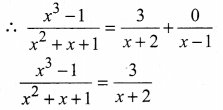## Tamilnadu Samacheer Kalvi 11th Maths Solutions Chapter 2 Basic Algebra Ex 2.9

Resolve the following rational expressions into partial fractions

11th Maths Exercise 2.9 Answers Question 1.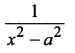Solution: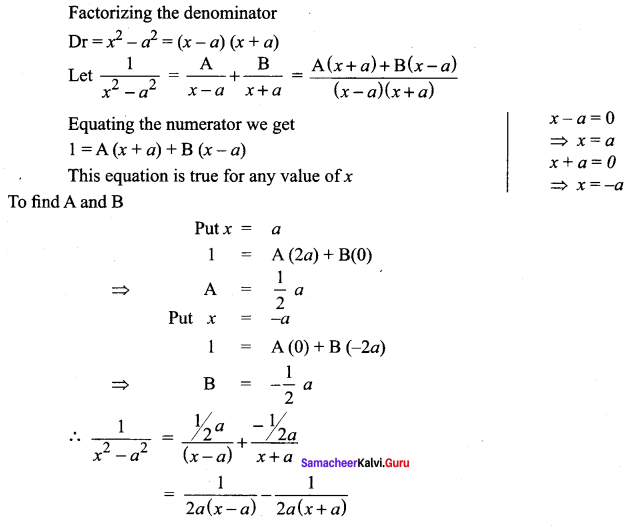11th Maths Exercise 2.9 Question 2.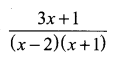Solution: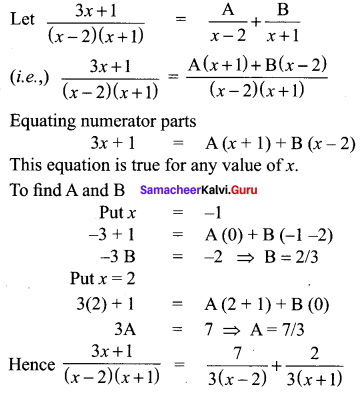Exercise 2.9 Class 11 Question 3.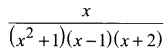Solution: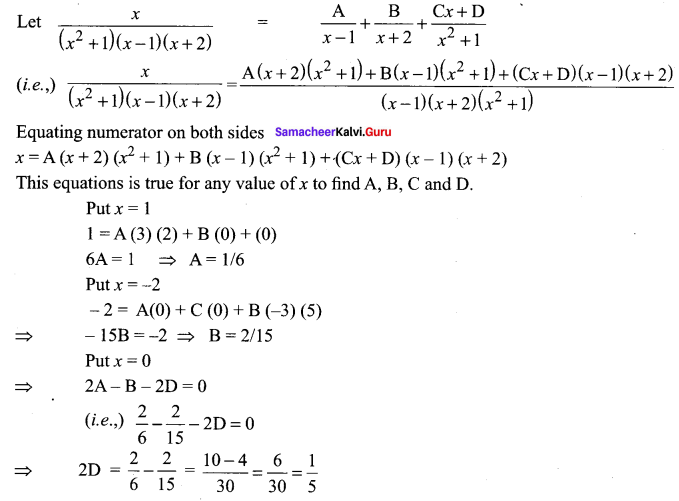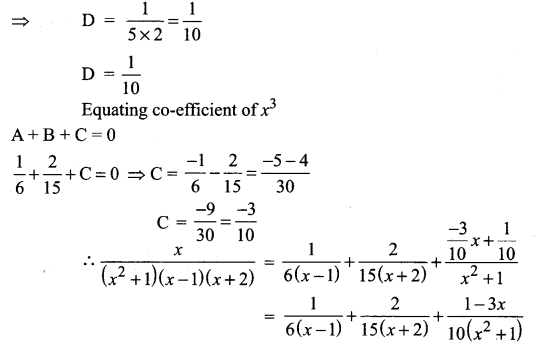11th Std Maths Exercise 2.9 Solutions Question 4.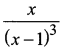Solution: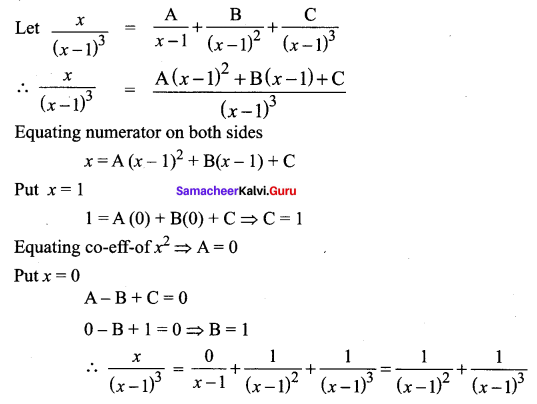11th Maths Exercise 2.9 Solutions Question 5.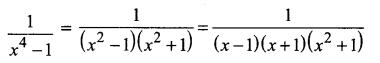Solution: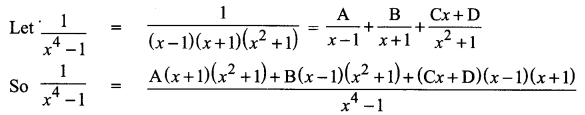Equating nuemarator on bothsides we get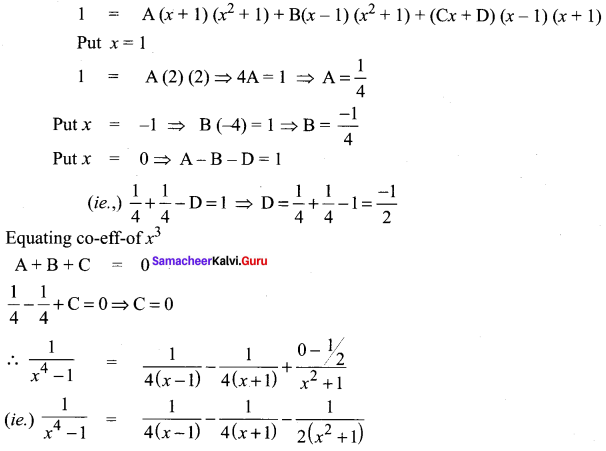Ex 2.9 Class 11 Maths Question 6.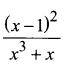Solution: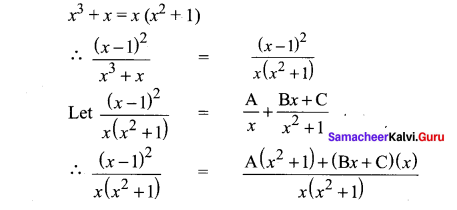Equating numerator on both sides
(x – 2)2 = A(x2 + 1) + (Bx + c)(x)
Put x = 0
1 = A
Equating co-eff of x2
1 = A + B
(i.e.,) 1 + B = 1 ⇒ B = 0
put x = 1
A(2) + B + C = 0 (i.e.,) 2A + B + C = 0
2 + 0 + C = 0 ⇒ C = -2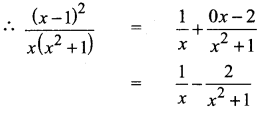11 Maths Exercise 2.9 Question 7.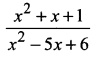Solution:
Since numerator and denominator are of same degree
we have divide the numerator by the denominator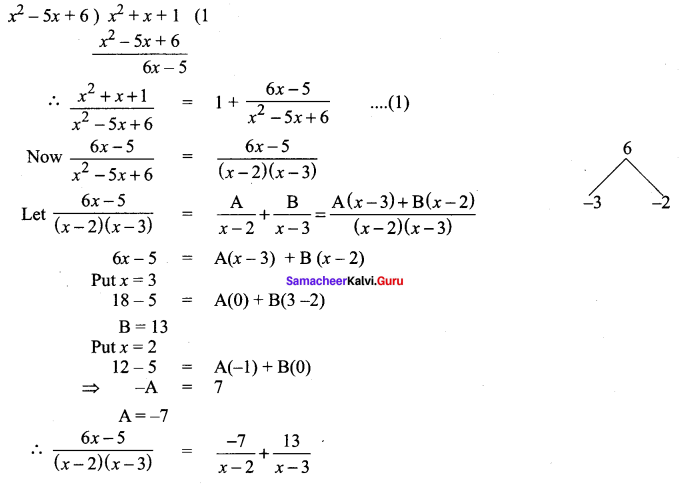Substituting the value in ….(1)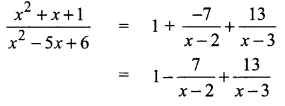Question 8.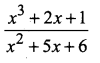Solution:
Numerator is of greater degree than the denominator
So dividing Numerator by the denominator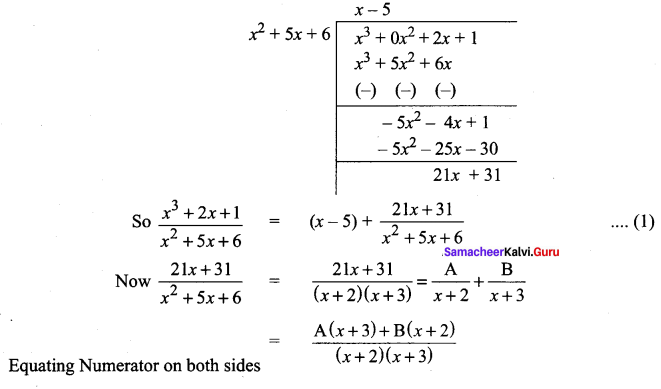⇒ 21x + 31 = A(x + 3) + B(x + 2)
Put x = -3
-63 + 31 = B(-1)
B = 32
Put x = -2
-42 + 31 = A(1) + B(0)
A = -11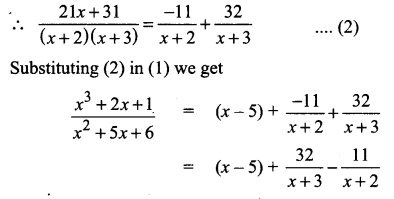11th Maths Exercise 2.9 3rd Sum Question 9.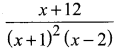Solution: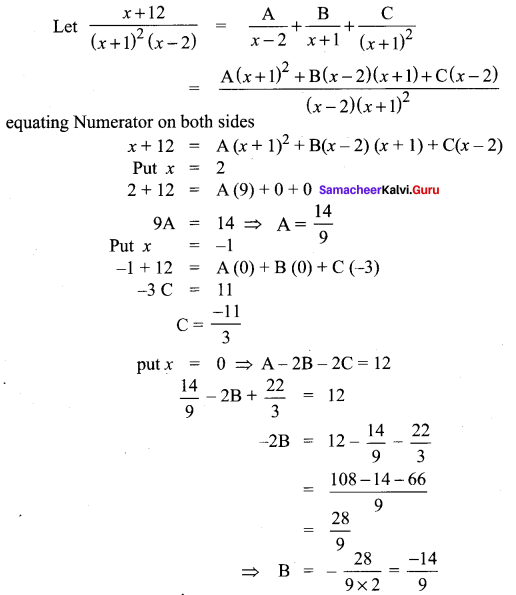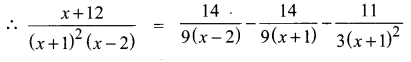11th Maths Exercise 2.9 5th Sum Question 10.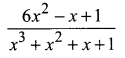Solution: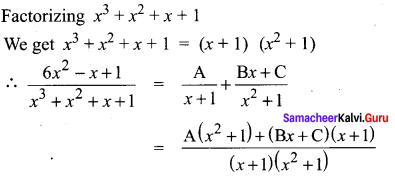Equating Numerator on both sides we get
6x2 – x + 1 = A(x2 + 1) + (Bx + c)(x + 1)
6 + 1 + 1 = A(2) + 0 ⇒ 2A = 8 ⇒ A = 4
Equating co-eff of x2
6 = A + B
(i.e.,) 4 + B = 6 ⇒ B = 6 – 4 = 2
put x = 0
1 = A+ C
4 + C = 1 ⇒ C = 1 – 4 = -3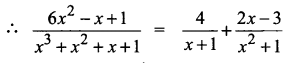Samacheer Kalvi 11th Maths Example Sums Question 11.Solution:
Since Numerator and are of same degree divide Numerator by the denominator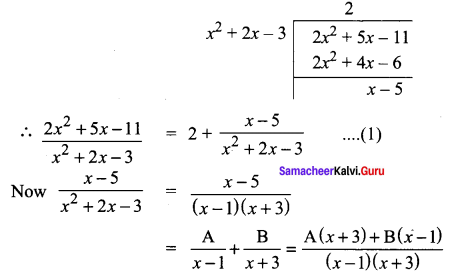equating Numerator on both sides we get
x – 5 = A(x + 3) + B(x – 1)
Put x = -3
-3 -5 = A(0) + B(-4)
-4B = -8 ⇒ B = 2
Put x = 1
1 – 5 = A(4) + B(0)
4A = -4 ⇒ A = -1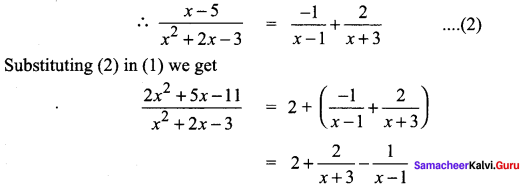Samacheer Kalvi Guru 11th Maths Question 12.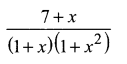Solution: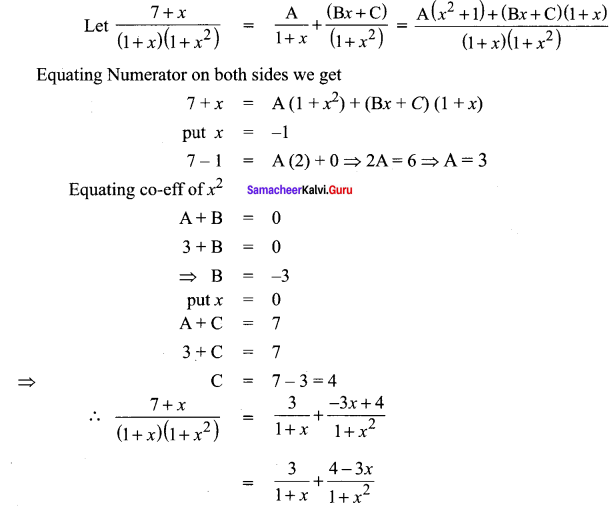### Samacheer Kalvi 11th Maths Solutions Chapter 2 Basic Algebra Ex 2.9 Additional Questions

Samacheer Kalvi 11 Maths Solutions Question 1.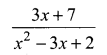Solution: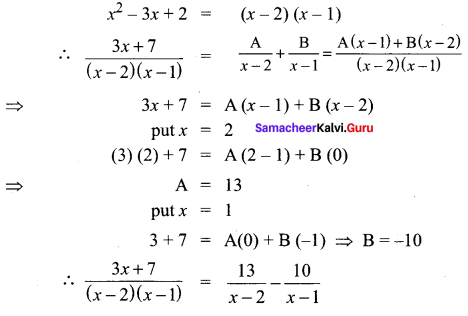Samacheer Kalvi 11th Maths Question 2.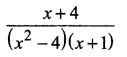Solution: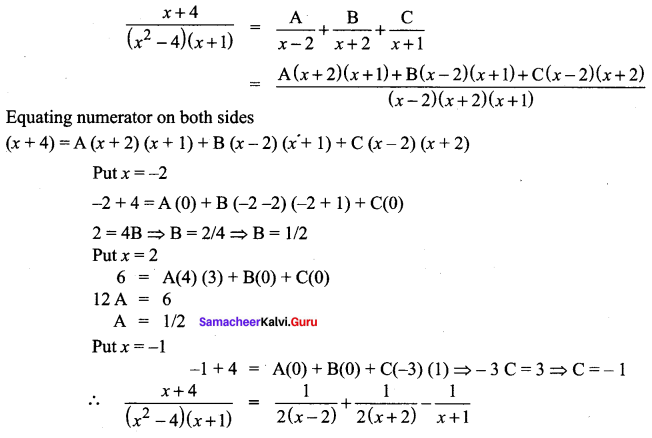Samacheer Kalvi 11th Maths Solution Question 3.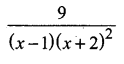Solution: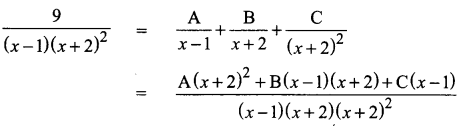Equating nemerator on b/s
9 = A(x+2)2 + B(x – 1)(x + 2) + C(x – 1)
Put x = -2
9 = A(0) + B(0) + C(-3)
-3C = 9 ⇒ C = -3
Put x = 1
9 = A (1 + 2)2 + B (0) + C(0)
9A = 9
A = 1
Put x = 0
9 = 4A – 2B – C
9 = 4(1) – 2B + 3
9 – 7 = -2B
2 = -2B
B = -1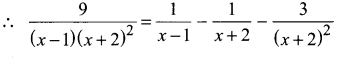Samacheer Kalvi 11th Maths Book Solutions Question 4.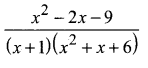Solution: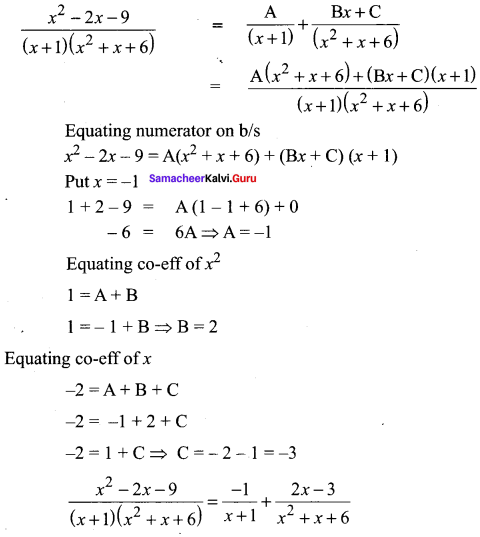11 Maths Solutions Samacheer Question 5.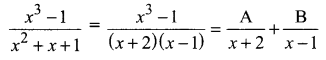Solution: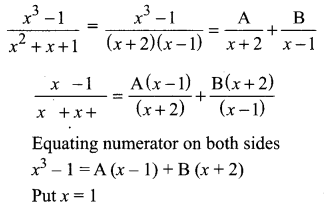0 = 0 + B(1 + 2)
3B = 0 ⇒ B = 0
Put x = -2
(-2)3 – 1 = A(-2 – 1) + B(0)
-8 – 1 = -3A
-9 = -3A
A = 9/3 ⇒ A = 3